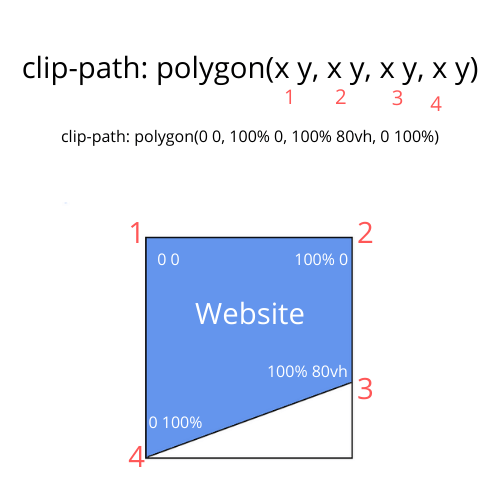# How to have a diagonal section in your website using css

Everyone is used to rectangular layouts and containers, so a well placed diagonal section can look really nice.

In this tutorial, I will show you how to create a diagonal layout using the clip-path property and also using skewY.

## Diagonal section using clip-path

One way of having a diagonal section is using `clip-path:polygon(x y, x y, x y, x y)`. Let me explain to you how it works.

We will be using `polygon(x y, x y, x y, x y)`. Think of a grid with its x and y coordinates. The first x and y correspond to the top left, the second to the top right, then bottom right, and finally, bottom left.Pretend you want a design like the one showed in the above screenshot. The top left is `0 0` because it is all the way at the start of the x-coordinate and the y-coordinate.

The top right is `100% 0`. x is 100% because it is all the way to the right of the x-coordinate. Since it is still all the way at the top then the y-coordinate remains at 0.

The bottom right is now `100% 80vh`. x = 100% because it is still all the way to the right of the x-coordinate. y is now 80vh because to get the slanted diagonal, we only want to go down to 80vh instead of the full 100%. If it was 100% for y then at the end we would just get a regular rectangular design.

Finally, the bottom left is `0 100%`. x is 0 because we are all the way back to the complete start. y is 100% because we want to go all the way to the bottom.

Of course, now that you know how the clip-path property works, you can manipulate the design and the slant as much as you want.

Here is a codepen showing how it works.

## Diagonal design using skew property

Another way to have a diagonal design is by using `transform:skewY(-10deg);`.

The way this works is you will skew the section you want to be diagonal. Let's say you skew it by -10deg. It would look like:

Now this is skewed too

The problem now is that the children are also all skewed. To fix this just unskew all the children by selecting all direct children and then skewY them back. So if you skewY the parent by -10deg then skewY the children by 10deg.

With SCSS, the code would be:

``````  & > * {
transform: skewY(10deg);
}
``````

or with css just do

``````.parent > * {
transform:skewY(10deg);
}
``````

Now it would look like this:

Now this is back to normal

We target all the direct children and unskew it by the same amount we skewed the section by.

Here is a codepen showing you the code.

I hope you now know how to make these diagonal layouts to add some cool designs to your websites.

Want to learn how to code and make money online? Check out CodingPhase (referral link)